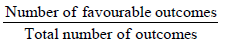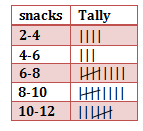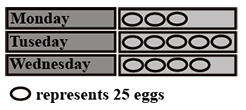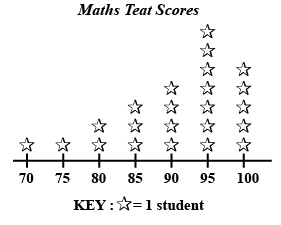Courses

# Points to Remember- Data Handling Class 8 Notes | EduRev

## Class 8 Mathematics by Full Circle

Created by: Full Circle

## Class 8 : Points to Remember- Data Handling Class 8 Notes | EduRev

The document Points to Remember- Data Handling Class 8 Notes | EduRev is a part of the Class 8 Course Class 8 Mathematics by Full Circle.
All you need of Class 8 at this link: Class 8

Facts That Matter

• The numerical information is called data.
• Data can be arranged and presented by grouped frequency distribution.
• Frequency is the number of times a particular entry occurs.
• Histogram is a special type of bar graph in which the class intervals are shown on the horizontal axis and heights of the bars correspond to the frequency of the class.
• In a histogram, there is no gap between bars.
• A circle graph or a pie chart shows the relation ship between a whole and its parts.
• Outcomes of an event or experiment are equally likely if each has the same of occurring.
• Probability of an event =• Probability of an event can have a value from 0 to 1.
• Probability of a sure event is 1.
• Probability of an impossible event is 0.

We Know That
Numerical information is called an observation. The collection of all these observations is called data. The collection of observation collected initially is called raw data. Raw data is tabulated in a precise manner. We may represent numerical data through pictures or graphs, which is called pictorial representation of the data. The pictorial representation of data using symbols is called pictograph. A display of information using bars of uniform width is called a bar-graph. In a bar-graph, the heights of bars are proportional to the respective values.

Solved Example:
Q: The table gives the number of snacks ordered and the number of days as a tally. Find the frequency of snacks ordered.Sol: From the frequency table the number of snacks ordered ranging between
2-4  is 4 days
4 to 6  is 3 days
6 to 8 is 9 days
8 to 10 is 9 days
10 to 12 is 7 days.
So the frequencies for all snacks ordered are 4, 3, 9, 9, 7

Q: The pictograph shows the number of eggs sold by a trader in three days. If the trader still had 115 eggs left after the three days, calculate the number of eggs he had at first.
a. 187
b. 425
c. 415
d. 98Sol:
The correct answer is “C”. Eggs Sold on the three days
Monday : 3 X 25 = 75
Tuesday: 5 X 25 = 125
Wednesday: 4 X 25 = 100
Eggs remaining = 115
Total number of eggs he had = 75 + 125 + 100 + 115 = 415

Q: The line plot below shows how students scored on last week’s math test. How many students scored 95 or higher on the test?
a. 5 students
b. 7 students
c. 11 students
d. 12 studentsSol: The correct answer is “D”. It is given that 1 star represents 1 student.

Students getting 95 marks = 7

Students getting 100 marks = 5

Therefore students getting 95 or above = 5 + 7= 12 students

93 docs|16 tests

,

,

,

,

,

,

,

,

,

,

,

,

,

,

,

,

,

,

,

,

,

;